# The Most Important Thing You Need to Know About Meta-Analyses

Publication
Article
Psychiatric TimesVol 39, Issue 10A busy clinician does not have time to read the vast number of randomized controlled trials (RCTs) published about different treatments. But good meta-analyses save us time by aggregating and summarizing data from relevant trials. Individual RCTs are sometimes small and suffer from methodological flaws. The results of RCTs are often influenced by sampling error. Good meta-analyses help us base decisions on larger numbers of subjects and reduce the effects of sampling error. To make the most of meta-analyses, we need to understand the most important information reported by a good meta-analysis. That information is the “prediction interval.” Although meta-analyses can focus on other topics, this article focuses on meta-analyses of treatments.

Using clear rules, a systematic review searches for studies on certain interventions for certain medical conditions. The authors of the study review the data from relevant studies to determine if helpful patterns can be found.

A meta-analysis is a systematic review that also includes a statistical synthesis of the data and calculation of overall, or “summary,” effect sizes. Thus, meta-analyses allow us to base treatment decisions on the best possible evidence determined from a thorough search for the evidence.

The summary effect tells us if a treatment’s average effect is clinically meaningful. But different patients and different populations of patients respond differently. Some patients will experience a below-average effect, some about an average effect, and some an above-average effect. Further, different studies report different average effect sizes. We need to know to what degree the effects are consistent or inconsistent. In meta-analysis, the degree of consistency or inconsistency is referred to as “dispersion” or “heterogeneity.”

Dispersion

Dispersion exists for 2 reasons: dispersion of true effects sizes and dispersion secondary to sampling error. True dispersion exists because different RCTs differ in at least 3 ways. First, different RCTs may include different subsets of a population (eg, 1 study may provide a particular drug to patients aged 18 to 64 years, and another RCT may provide the same drug to patients over aged 65 years). Second, RCTs are conducted according to different protocols (eg, 1 RCT may provide a form of psychotherapy for 12 sessions, and a different RCT may provide the same form of therapy for 20 sessions). Third, experimenters vary in their degree of skills in providing the treatment and in conducting the RCTs.

Dispersion due to sampling error occurs simply by chance. The sample drawn may not accurately reflect the population. For example, in a certain city, the population of individuals with depression may include 30% of patients who are 65 years and older. However, just by random chance, an experimental sample of patients with depression drawn from that city might consist of 45% of patients who are 65 years and older. If the RCT involves treating the patients with an antidepressant, the effect size may be reduced by the sampling error, given that older patients are less likely to respond to antidepressants.

We want to statistically eliminate the effect of sampling error and to know the dispersion of true effects. The prediction interval (PI) is the statistic that does this.

Prediction Interval

To fully explain the PI, we would have to see the equations and discuss them extensively. We do not have space for that, and the math is complex and requires many layers of computation. Instead, let’s introduce the concept and look at it graphically.Figure 1. Normally Distributed Study-Level Means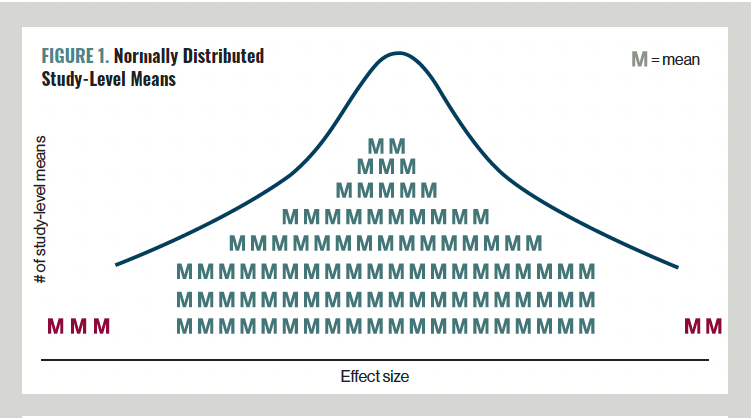The equation for the PI is based on the standard deviation of the mean effect sizes of the included studies. A PI is defined with a level of statistical significance, usually P = .05, in which case, we have a 95% PI. Assume we did 100 more studies. Every study would have its own mean effect size, m1, m2, m3, etc, all the way up to m100. Those 100 study-level mean effects sizes would vary from study to study. For a 95% PI, 95 of the studies would have a study-level mean effect size that fits somewhere between the low end and the high end of the PI. Five of the 100 studies would have a study-level mean effect size that is below or above the PI. If the study-level means were normally distributed, the distribution would look something like the graph in Figure 1. The blue curve represents the bell curve of a normal distribution of study-level means. The PI extends from the left end of the bell curve to the right end of the bell curve. There would be 95 green study-level means that fit within the PI and 5 red study-level means that fall outside the PI.Figure 2. Using the Prediction Interval in Clinical Practice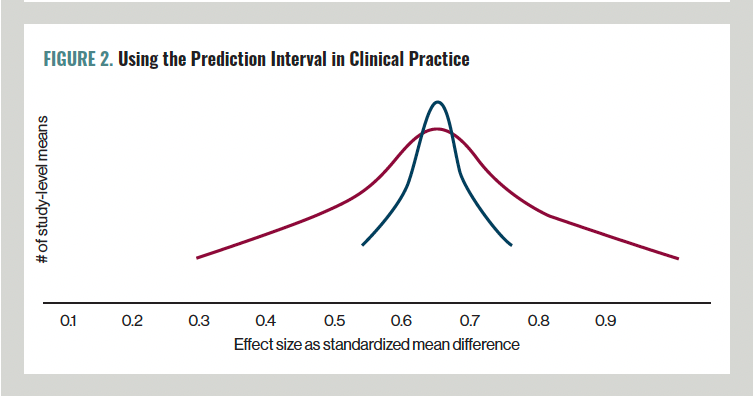Let’s continue to look at the PI graphically and see how we can use the PI in clinical practice. In Figure 2, let’s assume that the curves reflect different treatments and that each curve reflects the synthesis of a statistically sufficient number of studies—say 10 or more. Assume that the curves reflect dispersion of true effects because we have eliminated the effects of sampling error. That is, the curves illustrate normally distributed PIs. The green curve and the red curve have the same mean effect size. Assume that the average effect sizes are clinically meaningful at 0.65 on the X axis, but that any effect below 0.5 is not clinically meaningful. The red curve is much broader. The effects are highly dispersed and inconsistent (heterogeneous), and a substantial portion of the red curve extends below the clinically meaningful effect size of 0.5. If we did many studies, a considerable portion of them would find a true mean effect size below 0.5. If we provide the red treatment, there is a substantial chance our patient will not achieve improvement of at least 0.5.

In contrast, the green curve is much narrower. The results of the included studies are much more consistent. Even the leftward aspect of the green curve is above the clinically meaningful effect size of 0.5. If we provide the green treatment, we can be more confident that our patient will improve.Figure 3. Using the Prediction Interval to Choose Among 3 Treatments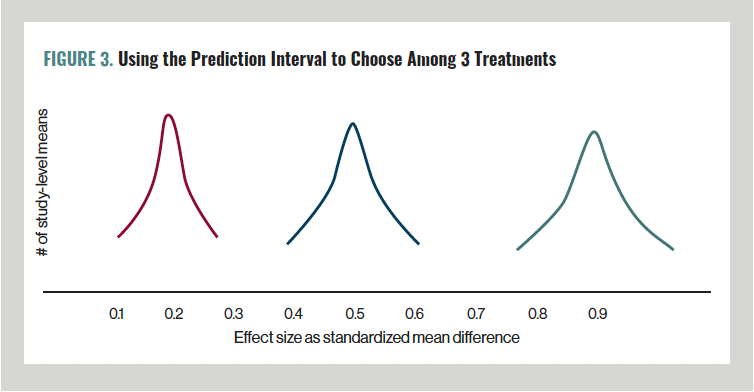In Figure 3, let’s look at some further examples to understand how we can use the PI. Again, assume that each curve represents the PI from a meta-analysis of 10 or more studies. Assume that the studies included substantial numbers of patients such as one for whom you need to develop a treatment plan. None of the red curve crosses over the clinically meaningful effect size of 0.5. This treatment is not likely to work. The blue curve straddles the clinically meaningful effect size. This treatment may or may not work. All of the green curve is above the clinically meaningful effect. This treatment is likely to work.

Of course, we cannot just look at numbers about treatment effects. In making clinical decisions, we also have to consider other factors, such as how serious the underlying medical condition is, the severity and probability of potential adverse effects, economic costs, all of those same factors about alternative treatments, and how likely it is that alternative treatments would work.

Prediction Interval vs Confidence Interval

Unfortunately, many—if not most—meta-analyses fail to report the PI. This omission makes it really hard to apply the results to clinical decision-making. Many, if not most, meta-analyses report the confidence interval (CI) and claim that this statistic represents dispersion or heterogeneity of true effect sizes. However, this is incorrect.Table. The Differences Between PI and CI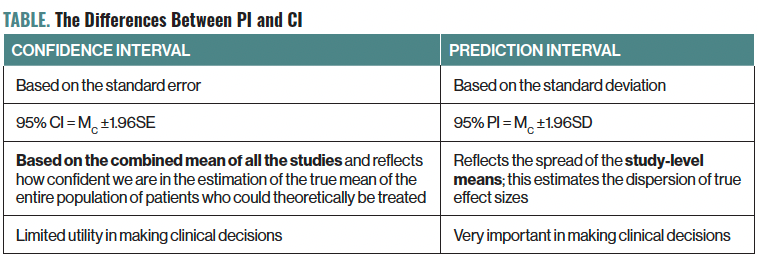Whereas the PI is calculated from the standard deviation, the CI derives from a different statistic: the standard error of the mean. The 95% PI = MC ±1.96SD, where MC is the mean effect of all the studies combined and SD is the standard deviation. The 95% CI = MC ±1.96SE where SE is the standard error. The CI reflects how confident we are in the estimation of the combined average effect size. We summarize the differences between the PI and the CI in the Table.Figure 4. Distinguishing Prediction Interval and Confidence Interval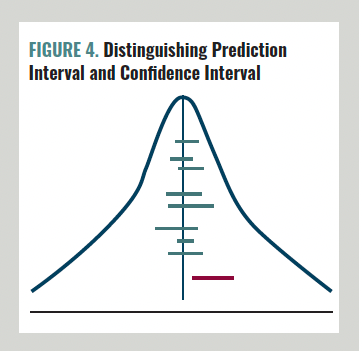Let’s look at this graphically as well. Imagine that our population of interest is everyone in a particular city with depression and that we treat every patient with depression in a placebo-controlled trial. Assume that the bell curve in Figure 4 illustrates the dispersion of the true effect size based on treating everyone in the population. Now imagine more realistically that we complete a series of studies of samples of patients from this population. A 95% CI means that if we did 100 studies, in 95 of those studies the CI would include the true mean of the entire population, but 5 of the CIs would not include the true mean. In Figure 4, the vertical line represents the true mean effect of the total population of individuals with depression in our city of interest. The horizontal lines represent the CIs of the individual studies. The CIs vary in width. If we did 100 studies, for 95 of them the CI would cross over the vertical line. Five of the CIs would not cross over the vertical line. In Figure 4, the green lines represent the 95 CIs that include the true mean effect size of the population. The red line represents the 5 CIs that do not include the true mean effect size of the population.

As the number of studies grows, the CI narrows and that means we are more confident in how well we have estimated the true mean effect of treating the entire population. But just being confident in the mean effect size does not tell us how dispersed or how consistent the effects are overall. To make clinical decisions, we want to know how broad the base of the bell curve is and where the bell curve fits compared with a clinically meaningful effect. The PI tells us this information.

Many, if not most, meta-analyses report the inconsistency statistic I2 (also called the heterogeneity statistic) and claim that this statistic represents dispersion or heterogeneity of true effect sizes. However, this is incorrect. I2 is not an absolute number. I2 is the following ratio: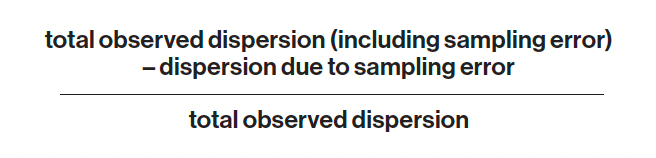An analogy might help us understand why we want to focus on an absolute amount and not a ratio. Imagine that you ask me, “What are your total financial assets?” I answer, “My house constitutes 25% of my total assets.” You asked about an absolute amount, but I answered in terms of a ratio. My house might be worth \$100,000, and my total assets might be \$400,000. Or my house might be worth \$500,000, and my total assets might be \$2,000,000. Either way, the ratio by itself does not answer your question about the absolute amount of my total assets.

I2 is reported as a percentage. According to different cutoff points, I2 is described as low (about 25%), medium (about 50%), or high (about 75%) heterogeneity. This practice is illegitimate and should be abandoned.

Concluding Thoughts

There is much more we could discuss about meta-analyses, but it is the PI that best informs clinical decision-making. Do not worry too much about the math. Use the PI intuitively. Determine what you believe is a clinically meaningful effect size, and then graph the PI. Then compare the curve of the PI to that of the meaningful effect size. Finally, estimate whether the treatment is likely to help your patient at issue.

For more information, see Borenstein et al, Introduction to Meta-Analysis, 2nd Edition.

Dr Moore is clinical associate professor of psychiatry at Texas A&M University College of Medicine and works at Baylor Scott & White Health Mental Health Clinic in Temple, Texas.